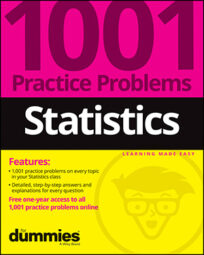##### Statistics: 1001 Practice Problems For Dummies (+ Free Online Practice)A confidence interval is a range of likely values for the population parameter. Suppose that you want to find the value of a certain population parameter (for example, the average gas price in Ohio). If the population is too large, you take a sample (such as 100 gas stations chosen at random) and use those results to estimate the population parameter.

Knowing sample results vary, you attach a margin of error (plus or minus), to cover your bases.

For the practice questions here, consider that: A clothing store is interested in the mean amount spent by all of its customers during shopping trips, so it examines a random sample of 100 electronic cash-register records and discovers that, among those who made purchases, the average amount spent was \$45 with a 95% confidence interval of \$41 to \$49.

## Sample questions

1. Which of the following are true statements regarding the 95% confidence interval for this data?

(A) If the same study were repeated many times, about 95% of the time the confidence interval would include the average money spent from the sample, which is \$45.

(B) If the same study were repeated many times, about 95% of the time, the confidence interval would contain the average money spent for all the customers.

(C) There is a 95% probability that the average money spent for all the customers is \$45.

(D) Choices (A) and (B)

(E) Choices (A), (B), and (C)

Answer: B. If the same study were repeated many times, about 95% of the time, the confidence interval would contain the average money spent for all the customers.

The average money spent for all the customers is an unknown value, called a population parameter. The average money spent for the 100 customers in the sample is a known value, \$45, which is called a statistic.

The store is using a sample statistic to estimate a population parameter. Because samples vary from sample to sample, they know the sample mean may not correspond exactly to the population mean, so they use confidence intervals to state a plausible range of values for the population mean.

If the same experiment were repeated many times (drawing a sample of the same size from the same population and calculating the sample average), the population mean would be expected to be contained in 95% of the confidence intervals created.

2. Which of the following is a reason for reporting a confidence interval as well as a point estimate for this data?

(A) The store studied a sample of sales records rather than the entire population of sales records.

(B) The confidence interval is certain to contain the population parameter.

(C) Because sample results vary, the sample mean is not expected to correspond exactly to the population mean, so a range of likely values is required.

(D) Choices (A) and (B)

(E) Choices (A) and (C)

Answer: E. Choices (A) and (C) (The store studied a sample of sales records rather than the entire population of sales records; because sample results vary, the sample mean is not expected to correspond exactly to the population mean, so a range of likely values is required.)

The store studied a sample of records to estimate a population parameter, and because sample results vary (called sampling error), the sample mean isn't expected to correspond exactly to the population mean. If another sample of the same size were drawn from the population, the sample mean would be expected to be somewhat different, so a range of likely values for the population mean (that is, a confidence interval) is required.

3. Which of the following statements is a valid argument for drawing a sample of size 500 rather than size 100?

(A) The larger sample will produce a less-biased estimate of the sample mean.

(B) The larger sample will produce a more precise estimate of the population mean.

(C) The 95% confidence interval calculated from the larger sample will be narrower.

(D) Choices (B) and (C)

(E) Choices (A), (B), and (C)

Answer: D. Choices (B) and (C) (The larger sample will produce a more precise estimate of the population mean; the 95% confidence interval calculated from the larger sample will be narrower.)

A larger sample drawn from the same population will tend to produce a narrower confidence interval and a more precise estimate of the population mean. The amount of bias isn't measured by the confidence interval.

If you need more practice on this and other topics from your statistics course, visit 1,001 Statistics Practice Problems For Dummies to purchase online access to 1,001 statistics practice problems! We can help you track your performance, see where you need to study, and create customized problem sets to master your stats skills.